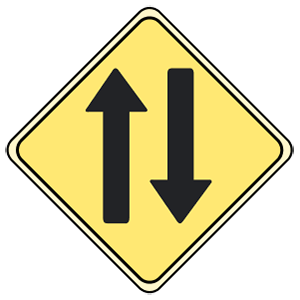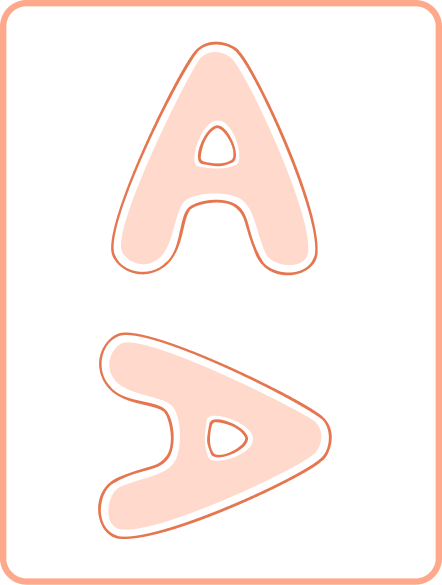# Clockwise – Definition With Examples

## What is Clockwise?

The clock’s name enlightens our mind with the image of a timepiece hanging on a wall, telling us what time it is. Although, did you know that the hands of one such timepiece can also give us a sense of direction? This is how the term “clockwise” came to be coined, denoting the direction of the movement of the hands of a clock.

We witness the clockwise (CW) direction on several occasions in our daily lives. From turning the knob of a door to the lid of a water bottle, we perform several daily activities which require us to rotate clockwise.

The CW direction is the opposite of the counterclockwise (CCW) direction, which indicates a turn to the left, instead of the right.

## Clockwise Definition

Clockwise (CW) refers to the direction of the hands of a clock. Since a clock is a mechanical machine that is meant to display time, its hands tend to move toward the right and leave a trail behind them, depicting what we refer to as the “clockwise” direction.

You may have observed how most screws are tightened, lids of bottles are closed or taps and faucets are turned off in the CW direction. Thus, CW is a term used to denote the rotational movement of an object.

Consider the movement of the hands of a clock. They move from the top to the right, then go down, turn left, and back up again. The opposite of CW movement is counterclockwise and depicts the opposite sense of rotation.

In the image shown above, the number “5” is rotated by 90 degrees CW. The movement does not affect the shape or size of the object, which means that even after CW rotation, the object’s symmetry remains the same.

## Measuring Angles of a Clockwise Rotation

Given a clock, there are 12 divisions with an angle of 30 degrees between each division. Every division is further divided into 5 equal parts, and the angular distance between each such part is 6 degrees. So, if we were to take the example of the digit “5” from above, we can say that the digit has moved 90 degrees CW.

## Solved Examples

Example 1: Taking the first figure as the original position of the penguin, identify which image out of (i) and (ii) shows a 90-degree CW turn.

Solution: The correct answer is the image (i).

The figure has moved from the original position toward the right, which is indicative of a CW movement.

Example 2: According to the map below, if X leaves their home and begins walking in a CW direction, where will they arrive first, the zoo or the store?

Solution: The correct answer is “store”.

According to the given map, if we assume that X leaves their home and starts walking in a CW direction, they will first come towards the art museum, then the store, and then the zoo, and if they continue to walk further, they will reach back home.

Example 3: Consider the letter “I”. If rotated CW by 90 degrees, which of the following will the letter denote?

(i) Horizontal Line

(ii) Vertical Line

Solution: The correct answer is a horizontal line.

Rotating the letter “I” in a CW manner will make it appear like a horizontal line.

## Practice Problems

1

### 1. In which direction has the arrow moved from the first position to the second?

Note: One turn will be equal to 90 degree rotation.One turn counterclockwise
One turn clockwise
Two turns clockwise
CorrectIncorrect
Correct answer is: Two turns clockwise
Since the arrow is facing the opposite direction from its original position, it has made two 90-degree turns in CW direction to reach the second position.
2

### A boy is facing north and turns 90 degrees in a CW direction. Which direction is he facing now?

East
West
South
North
CorrectIncorrect
After turning 90 degrees in the CW direction, the boy will turn to his right. Thus, from facing north, he will come to face the east direction.
3

### Determine the direction in which the alphabet has moved 90 degrees.Clockwise
Counterclockwise
Straight line
CorrectIncorrect